# Seminar 6

In this seminar, we will use Y.RData file that we created in the Seminar 2. We will practice exam-like questions on risk measure, and coding Value-at-Risk and Expected Shortfall for different volatility models. Please review the risk measures that we discussed in the lecture.

The plan for this week:

The following section is material for students to learn by themselves: Use a function to do historical simulation

## Practice exam questions on Risk Measures¶

### Question 1¶

Write down the mathematical definition of VaR (Value-at-Risk), and derive ES (Expected Shortfall).

#### Solution¶

VaR is a quantile on the distribution of P/L (profit and loss), so to write down the mathematical definition, we need to use $Q$, the random variable for the P/L on an investment portfolio. The realized value is $q$. So, we can denote the density of the P/L distribuition by $f_q(\cdot)$, and define VaR by:

$$\textrm{Pr}\ [Q\ \leq -\textrm{VaR}(p)] = p$$

or,

$$p = \int_{-\infty}^{-\textrm{VaR}(p)}f_q(x)dx$$

Expected shortfall is the expected loss conditional on VaR being violated:

$$\textrm{ES} = - E[Q|Q ≤ - \textrm{VaR(p)}]$$

If we write it down in its integral form:

$$\textrm{ES} = \int_{-\infty}^{-\textrm{VaR}(p)} x f_{\textrm{VaR}}(x)dx$$

The tail density, $f_{\textrm{VaR}}(\cdot)$ is given by:

$$1 = \int_{-\infty}^{-\textrm{VaR}(p)} f_{\textrm{VaR}}(x)dx\ =\ \frac{1}{p}\ \int_{-\infty}^{-\textrm{VaR}(p)} f_{q}(x)dx$$

### Question 2¶

What is a coherent risk measure?

#### Solution¶

Consider two real-valued random variables: $X$ and $Y$. A function $\varphi(\cdot): X,Y \rightarrow \mathbb{R}$ is called a coherent risk measure if it satisfies for $X$, $Y$, and constant $c$:

$\textrm{(a) Monotonicity}$ $$\textrm{if}\ X, Y \in V\ \textrm{and}\ X \leq Y,\ \textrm{then},\ \varphi(X) \geq \varphi(Y)$$

$\textrm{(b) Subadditivity}$ $$\textrm{if}\ X, Y, (X+Y) \in V,\ \textrm{then},\ \varphi(X+Y) \leq \varphi(X) + \varphi(Y)$$

$\textrm{(c) Positive homogeneity}$ $$\textrm{if}\ X \in V\ \textrm{and}\ c > 0,\ \textrm{then},\ \varphi(cX) = c\varphi(X)$$

$\textrm{(d) Translation invariance}$ $$\textrm{if}\ X \in V\ \textrm{and}\ c \in \mathbb{R},\ \textrm{then},\ \varphi(X + c) = \varphi(X) - c$$

### Question 3¶

Suppose you own two assets, A and B, with payoffs that are independent of each other. Each asset returns either 0 with probability 0.9, or -100 with probability 0.1. Is VaR(5%) sub-additive?

#### Solution¶

First let's consider only one of the assets, say asset A. With probability 10%, losses would be 100, and with probability 90%, losses would be 0. We can directly calculate the VaR of the single asset as:

VaR for A is: $$\textrm{VaR}^{5\%}(A) = 100$$ $$\textrm{VaR}^{15\%}(A) = 0$$

Now let's consider a owning one unit of asset A and one unit of asset B, so returns are (0.5A + 0.5B). We have a portfolio with two assets with independent payoffs. Each asset has two possible realizations, which means our portfolio has four possible payoffs. We can build a table with these payoffs and their corresponding probabilities:

Asset A Asset B Portfolio Return Probability
0 0 0 (0.9) * (0.9) = 0.81
-100 -100 -200 (0.1) * (0.1) = 0.01
0 -100 -100 (1 - 0.81 - 0.01) / 2 = 0.09
-100 0 -100 (1 - 0.81 - 0.01) / 2 = 0.09

If we order the possible portfolio returns and look for the 5% quantile, it will be the case where one asset incurs in a loss and the other does not. so $\textrm{VaR}^{5\%}(A+B) = 100$.

Hence:

$$\textrm{VaR}^{5\%}(A+B) = 100 \leq \textrm{VaR}^{5\%}(A) + \textrm{VaR}^{5\%}(B) = 200$$

### Question 4¶

Consider the asets in the last question. Is VaR(15%) sub-additive?

#### Solution¶

If we are considering a single asset, there is only a 10% probability of a loss of -100. This means that any VaR with probability larger than 10% will be equal to zero:

$$\textrm{VaR}^{15\%}(A) = \textrm{VaR}^{15\%}(B) = 0$$

For the case of the portfolio, ordering the possible returns in the table from Question 3 shows us that with a 15% probability, we would still be in the situation where one asset incurs in losses while the other does not, which means:

$$\textrm{VaR}^{15\%}(A + B) = 100$$

Since the VaR of the portfolio is larger than the sum of the individual VaRs, VaR(15%) fails to meet subadditivity.

### Question 5¶

Give one example of a traded asset that could lead to a sub-additivity violation of VaR, carefully explaining why the payoff structure of this asset would lead to that conclusion.

#### Solution¶

An asset that most of the time has a return of zero, but occasionally very negative. This could be the exchange rate of a country with a peg, that occasionally devalues, short deep out of the money option or junk bonds.

The payoff structure of these type of assets can resemble the one discussed in Question 3, which means that by diversifying, you can have a positive VaR for a probability that would give you a VaR of 0 if you were considering a single asset.

### Question 6¶

Suppose you own 1 million USD worth of both stocks A and B. Stock A is a small cap stock, with a market capitalization of 3 million, while stock B is a large cap stock, with a market capitalization of 3 billion. As a consequence, one of the axioms of a coherent risk measure is likely to be violated for VaR on one of the stocks but not the other. Which stock is it and why would that be the case?

#### Solution¶

This question is about the positive homogeneity property of risk measures: $$c \times Risk(A) = Risk(c\times A)$$ for an asset $A$ and constant $c$.

It is very different owning 1 million USD worth of a stock with a total market capitalization of 3 million, or a stock with total market capitalization of 3 billion. In the first case, we have a big influence on the price of the stock. If we decide to sell all our holdings, the price will go down as we do. This means we will not be getting the same amount of money for each dollar worth of stock we sell. In the case of the large capitalization company, our holdings are a small share of the total (less than 1%), so it is unlikely that any transaction we do will have a large impact on the stock price.

So, positive homogeneity is likely to hold for the big capitalization firm, but not for the small one.

### Question 7¶

State the three steps in VaR calculations.

#### Solution¶

(a) To specify probability $p$. Commonly used are VaR levels of 1% - 5%, but it is mainly determined by how the risk managers wishes to interpret the VaR number.

(b) To decide holding periods, commonly 1 day, but it can vary depending on different circumstances.

(c) Identification of probability distribution of the profit and loss of the portfolio.

### Question 8¶

Derive the VaR for simple returns and holding one stock

#### Solution¶

The definition of simple returns is $$R_{t} = \frac{P_{t} - P_{t-1}}{P_{t-1}}$$ The definition of VaR is

($Q_{t}$ is the random variable for the P/L on a stock)

\begin{eqnarray} p = Pr(Q_{t} \leq -VaR(p)) = Pr(P_{t} - P_{t-1} \leq -VaR(p)) = Pr(P_{t-1}R_{t} \leq -VaR(p)) = Pr(\frac{R_{t}}{\sigma} \leq - \frac{VaR(p)}{P_{t-1}\sigma}) \end{eqnarray}

Denote the distribution of sandarized returns by $F_{R}()$. Then we have $$VaR(p) = - \sigma F^{-1}_{R}P_{t-1}$$

### Question 9¶

State the some issues for VaR

#### Solution¶

(a) VaR is not a coherent risk measure (not subadditive)

(b) VaR is easy to manipulate

(c) VaR is only a quantile on the P/L distribution

(d) VaR does not measure the worst case of loss

## Estimating VaR and ES with historical simulation - Univariate¶

Historical simulation is a non-parametric method to implement risk measures. It assumes that the past is a sufficient predictor for the future, so in other words, that "history repeats itself". It uses the quantiles of the empirical disitribution of returns. The procedure to implement it for estimating VaR is:

• Decide on a probability, $p$, e.g. 1%
• Have a sample of returns, $y$, with length $T$, e.g. 1000 days (for multi-assets, you can treat $y$ as the portfolio returns)
• Sort the $y$ from smalles to largest, call that $ys$
• Take the $(T \times p)^{th} = (1000 \times 0.01)^{th}$ smallest value of $ys$, call that $ys_{T \times p} = ys_{10}$
• If you own one stock, and $P_{t-1}=1$, then VaR is the $10^{th}$ smallest return, i.e.
$$VaR_t = -ys_{10}$$
• Otherwise, you have to multiply that by the number of stocks you own, and their $t-1$ price:
$$VaR_t = -ys_{10} P_{t-1} \times \textrm{number of stocks}$$

To implement it for ES:

• Take the mean of all observations that violate VaR
• For example, for the previous case:
$$\textrm{ES} = \frac{1}{10} \sum_{i=1}^{10} ys_i$$

#### Portfolio Value¶

We will use the term portfolio value to denote the monetary value of a portfolio. For example, if we own 100 stocks, each worth 10 USD, the value of our portfolio is of 1000 USD. We can extend this idea to a portfolio with different assets. If we have 5 stocks of A, each worth 10 USD, and 20 stocks of B, each worth 2 USD, the value of our portfolio is: (5)(10) + (20)(2) = 90 USD.

### Implementing VaR and ES for General Electric¶

We will use historical simulation to esimate VaR and ES for the returns of General Electric:

In :
# Implementing HS to estimate VaR and ES

# Extract the returns for GE
y <- Y$GE # View them head(y)  1. 0.034289343141517 2. -0.00187475625782325 3. -0.00564490252545496 4. -0.00947878204978265 5. 0.00569773702368828 6. -0.0210530687723584 In : # Plot the returns plot(y, type = "l", main = "Returns for GE")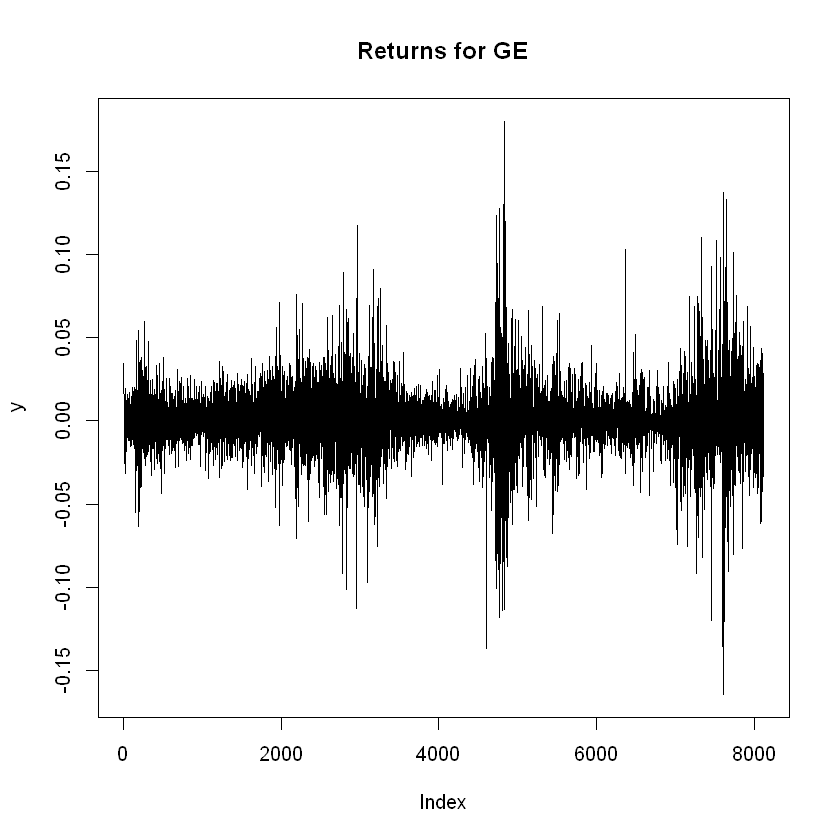In : # Specify the probability p p <- 0.05 # Assume we have a portfolio value of 1000 USD portfolio <- 1000 # Sort the values in y using sort() ys <- sort(y) # Plot them plot(ys, type = "l", main = "Sorted returns for GE", las = 1)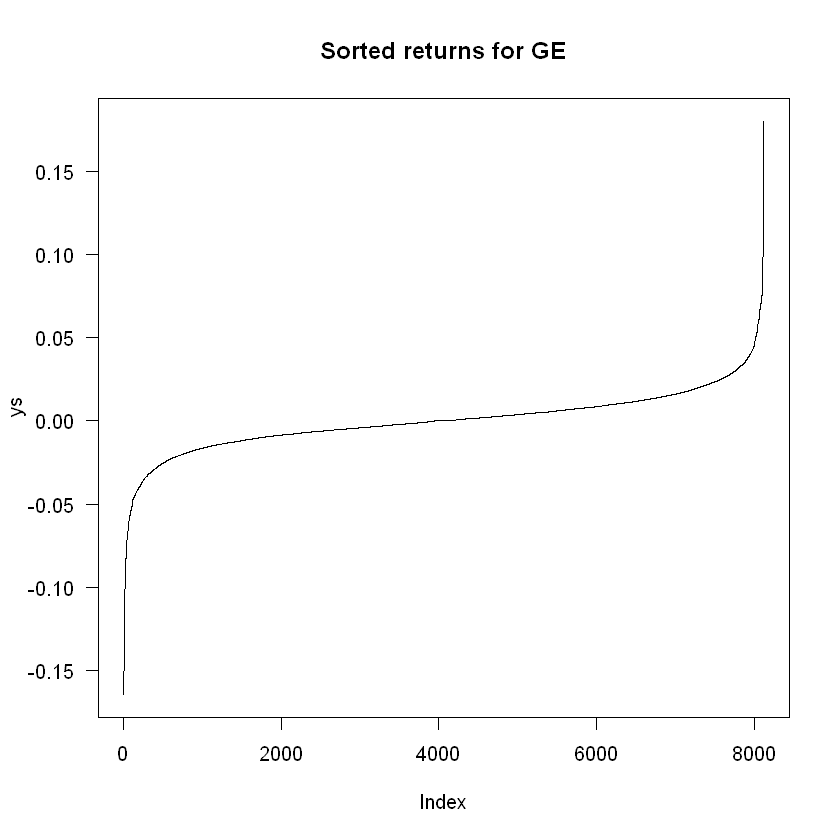In : # Number of observations n <- length(y) # Get the 5% quantile by multiplying the length times p n * p  406.3 Since this number is not an integer, it is a good practice to round it up. If we round it down, we are not meeting the required probability, since the element in the 406th position has a quantile lower than 5%. By rounding up and using the element in the 407th position, we guarantee to be covering the 5% quantile. We can use the function ceiling() for this: In : # Round up quant <- ceiling(n*p) quant  407 Now we search for the 407th element in the sorted vector ys: In : # Find the 407th element in ys ys[quant]  -0.0285404309576472 We can visually see this in the plot for the sorted list: In : # Visually finding the 5% quantile plot(ys, type = "l", main = "Sorted returns for GE", las = 1) # Adding the segments segments(x0 = quant, y0 = -0.5, y1 = ys[quant], lty = "dashed", lwd = 2, col ="red") segments(x0 = -1000, y0 = ys[quant], x1 = quant, lty = "dashed", lwd = 2, col ="red") # Adding ticks axis(1, at = quant, label = quant) axis(2, at = ys[quant], label = round(ys[quant],3), las = 1)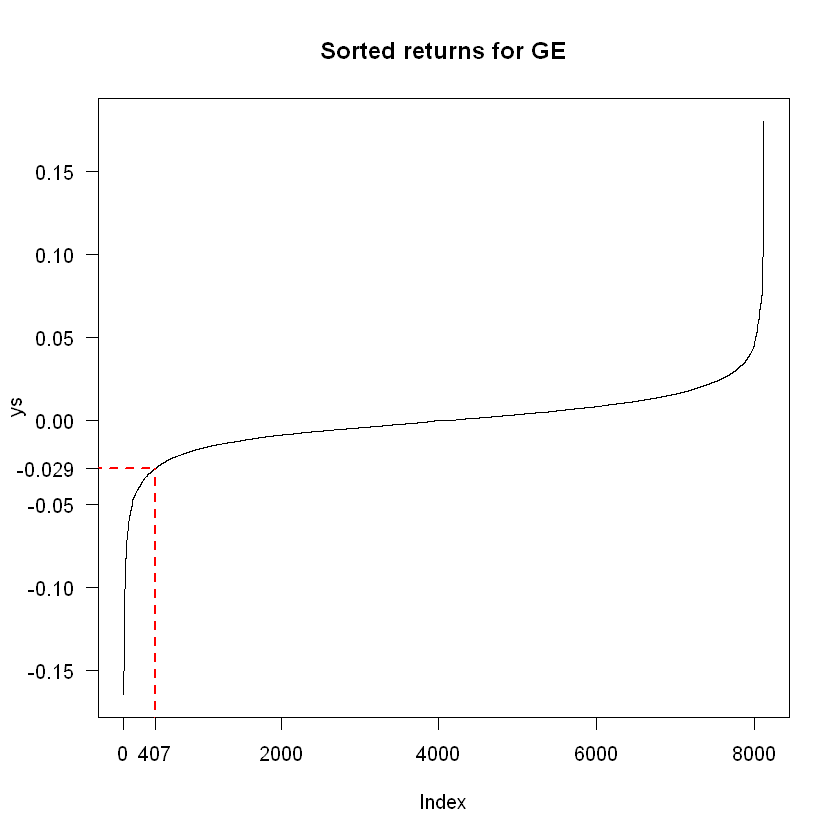In order to calculate VaR, we multiply this return by the portfolio value, with a minus sign in front since it is a loss: In : # Use it to calculate VaR, scaling for the portfolio value VaR <- -ys[quant] * portfolio VaR  28.5404309576472 To calculate the ES, we take the mean of returns conditional on VaR being violated, and scale this to the portfolio size. In other words In : # For ES, we get the mean of observations up to the 5th quantile ES <- -mean(ys[1:quant]) * portfolio ES  45.8079378388257 In : # Report our findings: cat("We have", length(y), "observations on GE", "\n", p , "VaR is", VaR, "and ES is", ES, "on portfolio worth$", portfolio, "\n")

We have 8126 observations on GE
0.05 VaR is 28.54043 and ES is 45.80794 on portfolio worth $1000  A direct way to find the quantile of an empirical distribution is using the quantile() function on the returns. But bear in mind that if the quantile index is not integer, it will output a weighted average between the rounded-up and rounded-down values. By calling quantile(y,0.05) we see a small difference: In : # Doing HS with the quantile() function quant2 <- quantile(y,0.05) quant2 # VaR VaR <- -quant2*portfolio cat("5% VaR is:", VaR)  5%: -0.028536057951545 5% VaR is: 28.53606 ## Estimating VaR and ES with historical simulation - Multivariate¶ The multivariate extension to HS is quite straightforward. We use the matrix of returns and a vector of weights to create a time series vector of portfolio returns, and treat it as if it were a single asset. In this section we will estimate VaR through HS for General Electric and Citigroup: In : # HS for GE and C # Get the returns from GE and C and transform into a matrix y <- as.matrix(Y[,c("GE", "C")]) # Create a vector of portfolio weights, here we will split the stocks halfway w <- c(0.5, 0.5) # Multiply the matrix y (dimension Tx2) by the vector w (dimension 2x1) # Output yp is portfolio returns, dimension (Tx1) yp <- y %*% w head(yp)   0.0322647 0.00540522 -0.00916501 -0.000501898 0.00705066 -0.0168423 In : # Plot the portfolio returns plot(Y$date, yp, main = "Portfolio returns for GE and C", type = "l", las = 1, lwd = 2, col = "red")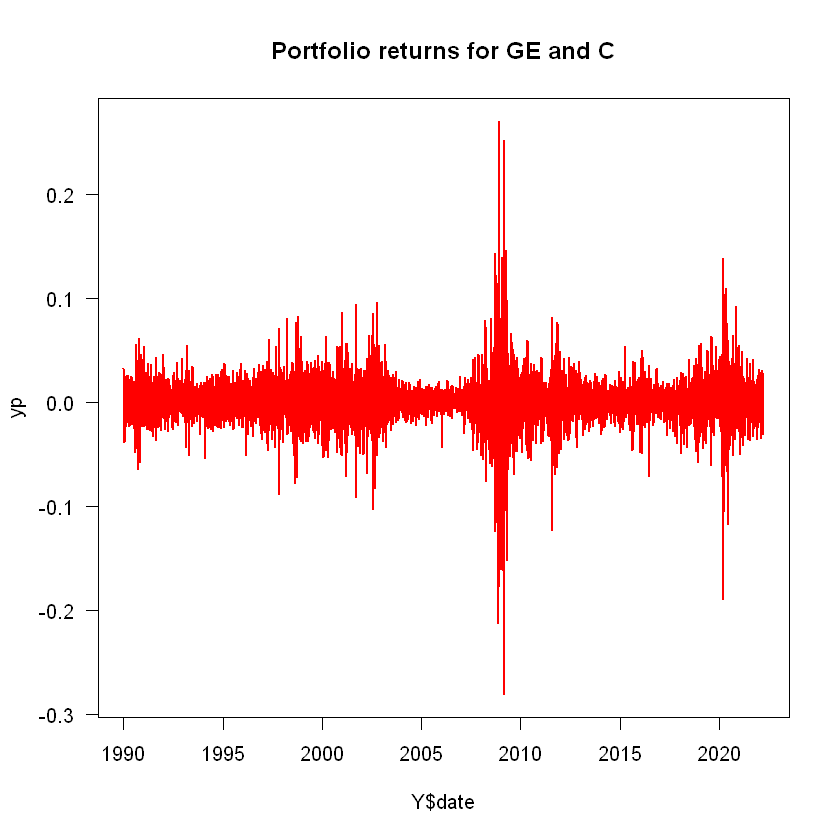In :
# Get VaR and ES

# Sort the vector
ys <- sort(yp)

# Get the length
n <- length(ys)

# Get the position of 5% quantile
quant <- ceiling(n*p)

# VaR
VaR <- -ys[quant]*portfolio

# ES
ES <- -mean(ys[1:quant]) * portfolio

cat("We have", length(ys), "observations on the portfolio", "\n",
p , "VaR is", VaR, "and ES is", ES, "on portfolio worth $", portfolio, "\n")  We have 8126 observations on the portfolio 0.05 VaR is 28.44304 and ES is 48.90217 on portfolio worth$ 1000


## Historical simulation VaR with different estimation windows¶

### Out-of-sample forecasting and estimation windows¶

We usually use a fixed estimation window to do a one-day-ahead VaR forecast recursively. For example, we can the data from day 1 to day 1000 to forecast VaR for day 1001. This is an out-of-sample forecast, since the data we are using only comprises days 1 to 1000, but we are doing a forecast for day 1001, which is not in our sample. Then we will move one period ahead and use data from day 2 to day 1001 to forecast VaR for day 1002 and so on. The estimation window, denoted by $W_E$ is how many days we include in the sample to forecast for one day ahead. In the example just mentioned, the estimation window is 1000 days.

Assume we have a total of $T$ data points. After we are doing with this recursive one-day-ahead forecasting, we will have made $T-W_E$ forecasts.

### Implementation¶

Let's work again with the returns of General Electric and use a fixed estimation window of 1000 days. We will need to implement a for-loop to get the VaR estimation for each day between days 1001 and the length of the dataset.

In :
# Historical simulation with fixed estimation window
y <- Y$GE window <- 1000 p <- 0.05 n <- length(y) portfolio_value <- 1000 # Initialize and empty vector to hold VaR forecasts VaR <- vector(length = (n - window)) # Loop to calculate one VaR per day for (i in 1:(n-window)) { ys <- sort(y[i:(i+window)]) quant <- ceiling(p*length(ys)) VaR[i] <- -ys[quant]*portfolio_value }  In : # Plot it plot(VaR, type = "l", main = "VaR HS with Estimation Window 1000", col = "red", las = 1, ylab = "USD")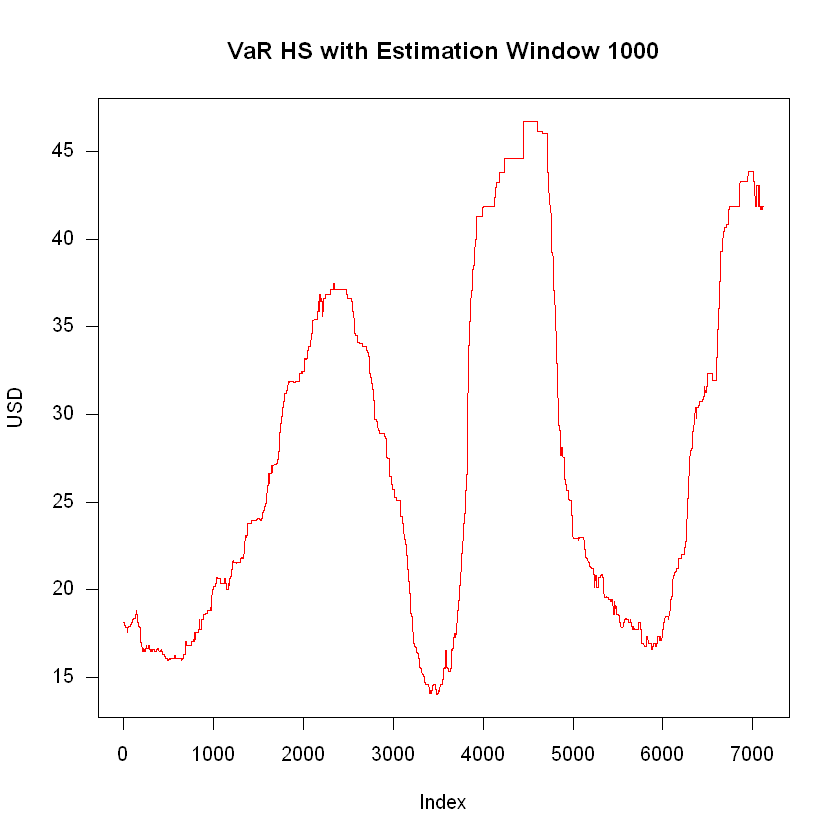In : # We should add the dates in the x-axis # Notice we have to exclude the first 1000 days dates_var <- Y$date[1001:length(Y$date)] # Plot again plot(dates_var, VaR, type = "l", main = "VaR HS with Estimation Window 1000", col = "red", las = 1, ylab = "USD", xlab = "Date")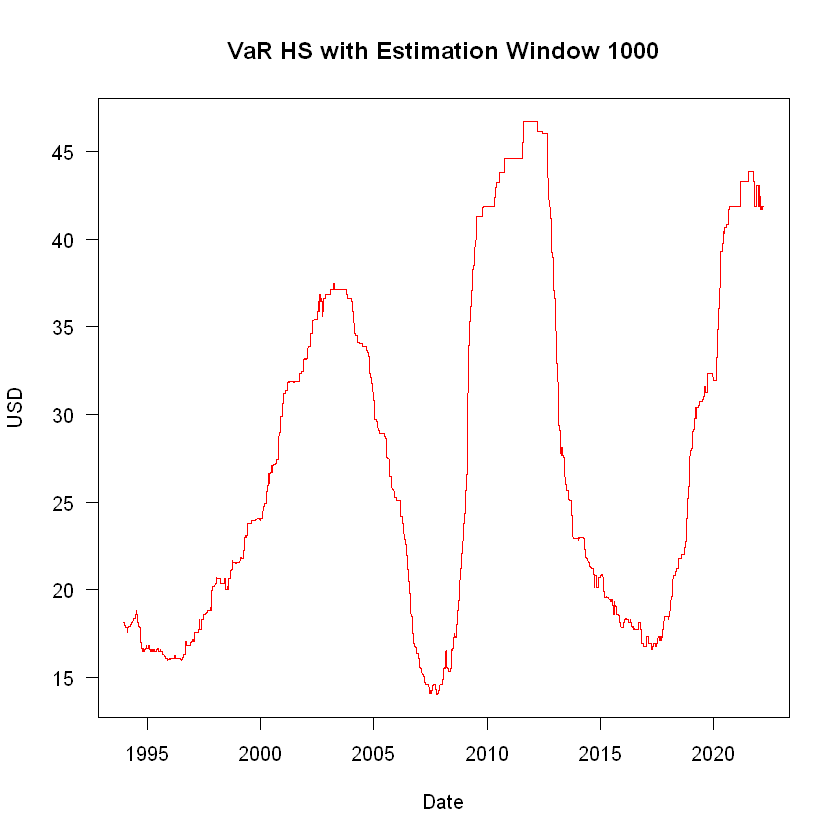### Different Estimation Windows¶ We have used a fixed estimation window of 1000 days. To see the effect of changing the estimation window, we will reproduce this exercise using estimation windows of 100, 500, 1000 and 5000 days: In : windows <- c(100, 500, 1000, 5000) which(windows == 100)  1 In : # Using four different estimation window sizes windows <- c(100, 500, 1000, 5000) # Create an empty data frame to fill with the forecasts HS <- data.frame(HS100 = numeric(), HS500 = numeric(), HS1000 = numeric(), HS5000 = numeric()) # Do a loop for every element of windows for (window in windows) { # Perform a daily HS VaR for (i in 1:(n-window)) { # Sort the returns for the respective estimation window ys <- sort(y[i:(i+window)]) # Get the quantile quant <- ceiling(p*length(ys)) # Allocate the result to the corresponding column of HS # Use which() to find the index of the window and allocate to that column column <- which(windows == window) HS[i+window, column] <- -ys[quant]*portfolio_value } }  Now that we have implemented HS VaR for four different estimation windows, we can compare them. We need to be careful in this step because each model has a different number of forecasts, given that the number of observations used is different. We should always focus on the time frame of the most restrictive model, in this case, the one using an estimation window of 5000 observations. Let's plot all of them together: In : # Plotting the forecasts plot(HS$HS100, main = "HS with different estimation windows", ylab = "VaR in USD",
xlab = "Date", type = "l", col = "red", lwd = 2)
lines(HS$HS500, col = "blue", lwd = 2) lines(HS$HS1000, col = "green", lwd = 2)
lines(HS$HS5000, col = "black", lwd = 2) legend("topright", legend = names(HS), lty = 1, col = c("red", "blue", "green", "black"))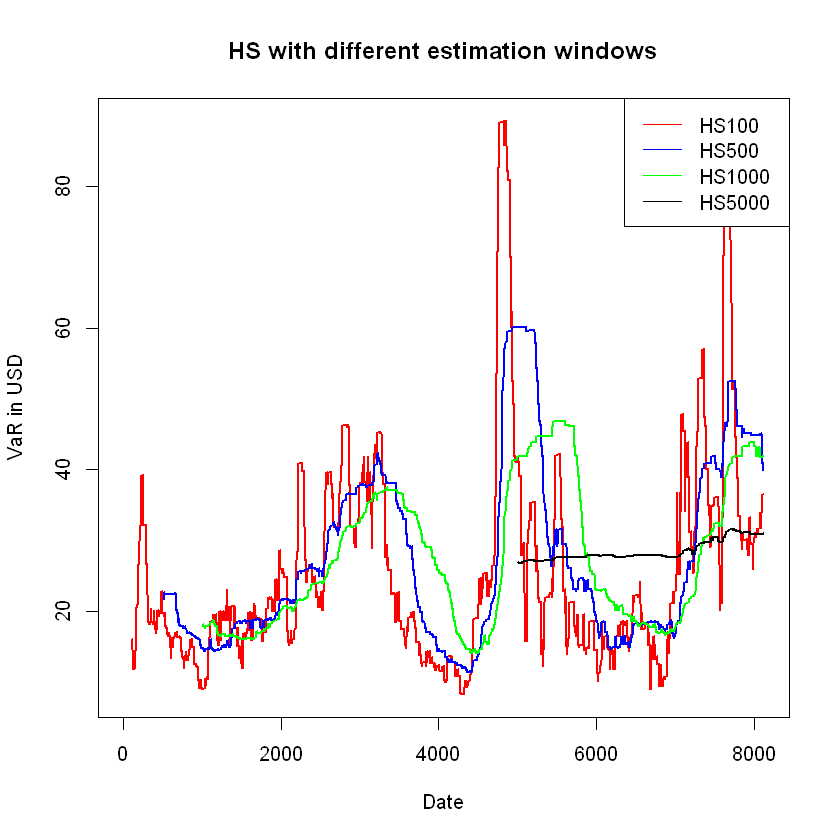For a better comparison, let's restrict our attention to the time frame where we have forecasts for each model. This consists of the length of the data minus the largest estimation window. Also, we will add dates: In : # Subset HS to keep only the observations that fit the most restrictive estimation window HS <- HS[(max(windows)+1):n,] # Extract the dates to use dates <- Y$date[(max(windows)+1):n]

# Plotting them all together
plot(dates, HS$HS100, main = "HS with different estimation windows", ylab = "VaR in USD", xlab = "Date", type = "l", col = "red", lwd = 2) lines(dates,HS$HS500, col = "blue", lwd = 2)
lines(dates,HS$HS1000, col = "green", lwd = 2) lines(dates,HS$HS5000, col = "black", lwd = 2)

legend("bottomright", legend = names(HS), lty = 1, col = c("red", "blue", "green", "black"))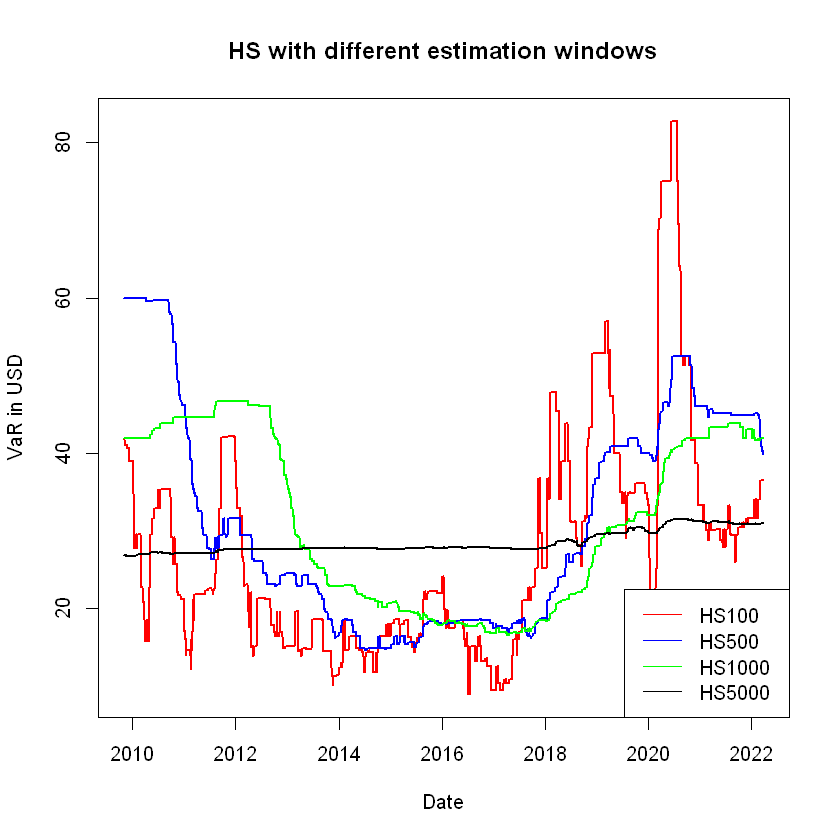Let's compute the means for each forecast:

In :
# Means
colMeans(HS)

HS100
27.3015189102817
HS500
31.145162410714
HS1000
31.2305923363665
HS5000
28.4374691948126

And the standard deviations:

In :
# Standard deviations
sd(HS$HS100) sd(HS$HS500)
sd(HS$HS1000) sd(HS$HS5000)

14.3960848724283
14.0419016013412
11.0932423710107
1.39300470685819

Intuitively, the most extreme observations, which are the ones that determine our forecast, fluctuate a lot more than observations that are less extreme. so, the bigger the sample size used as estimation window, the more precise the estimation of HS VaR should be. However, the downside is that old data may not be at all representative. In the plot we see that with an estimation window of 5000 days, the forecast is basically flat, and it fluctuates more the smaller the estimation window is, even if the mean does not vary much. ___

## Use a function to do historical simulation (Not covered in class)¶

When implementing HS VaR for different estimation windows, the best way to proceed is to create a function that takes a dataset, a probability, a portfolio value, an estimation window, and builds a forecast:

In :
# Creating a HS VaR forecast function

DoHS <- function(y, probability = 0.05, portfolio_value = 1, WE = 1000){
# HS function that takes as argument:
# y: A vector of returns, ordered by date
# probability: The probability to be used for VaR - Default 5%
# portfolio_value: The portfolio value - Default 1
# WE: Estimation window for the forecast - Default 1000 days

# To calculate elapsed time, first get the current time
old <- Sys.time()

# Print message
cat("Doing Historical simulation VaR forecast", "\n",
"Estimation window:", WE, "\n",
"Number of observations:", length(y), "\n",
"VaR probability:", probability, "\n",
"Portfolio value:", portfolio_value)

# Number of observations
n <- length(y)

# Initialize empty VaR vector
VaR <- rep(NA, n)

# Do a loop for the forecast
for (i in 1:(n-WE)){

# Sort the returns for the estimation window
ys <- sort(y[i:(i+WE-1)])

# Get the quantile position, using ceiling to round up
quant <- ceiling(probability * length(ys))

# Allocate the VaR forecast in the vector
VaR[i+WE] <- -ys[quant]*portfolio_value
}

# Get the new time and print the elapsed time
time <- Sys.time() - old
cat("\n", "Elapsed time:", round(time,4), "seconds")

# Return the VaR vector
return(VaR)
}


Now we can use DoHS() to automatically create a HS VaR forecast for the estimation window we specify.

Let's do a forecast using the estimation windows of 300, 1000 and 2000 days for the returns of Microsoft:

In :
# Loading the returns for Microsoft
y <- Y$MSFT dates <- Y$date

# Plot the returns
plot(dates, y, main = "Microsoft returns", type = "l", lwd = 2, las = 1,
xlab = "Date", ylab = "Returns")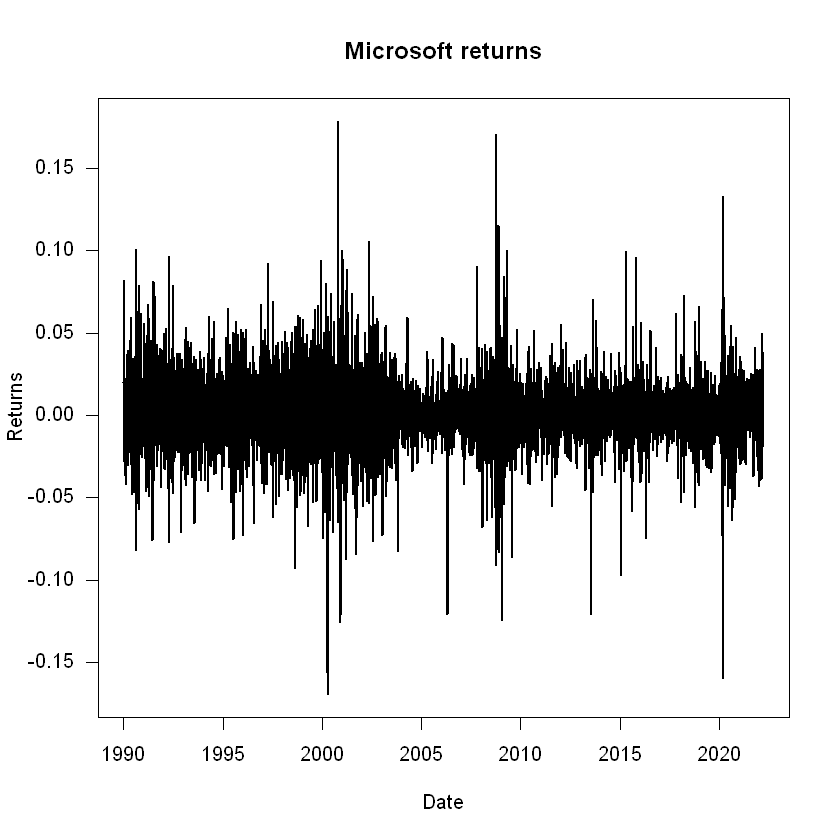In :
# Historical Simulation with an estimation window of 300 days
HS300 <- DoHS(y, probability = p, portfolio_value = portfolio_value, WE = 300)

Doing Historical simulation VaR forecast
Estimation window: 300
Number of observations: 8126
VaR probability: 0.05
Portfolio value: 1000
Elapsed time: 0.4096 seconds
In :
# Historical Simulation with an estimation window of 1000 days
HS1000 <- DoHS(y, probability = p, portfolio_value = portfolio_value, WE = 1000)

Doing Historical simulation VaR forecast
Estimation window: 1000
Number of observations: 8126
VaR probability: 0.05
Portfolio value: 1000
Elapsed time: 0.5303 seconds
In :
# Historical Simulation with an estimation window of 2000 days
HS2000 <- DoHS(y, probability = p, portfolio_value = portfolio_value, WE = 2000)

Doing Historical simulation VaR forecast
Estimation window: 2000
Number of observations: 8126
VaR probability: 0.05
Portfolio value: 1000
Elapsed time: 0.7077 seconds

We can plot the three forecasts using matplot():

In :
# Bind HS into a matrix
HS_VaR <- cbind(HS300, HS1000, HS2000)

# Plot and modify axis to include dates
matplot(dates, HS_VaR, type = "l", lty = 1, col = 1:3, xaxt = "n", main = "HS VaR", xlab = "Date", ylab = "VaR USD")
axis.Date(1, at = seq(min(dates), max(dates), by = "years"))

# Legend
legend("bottomleft", legend = colnames(HS_VaR), lty = 1, col = 1:3)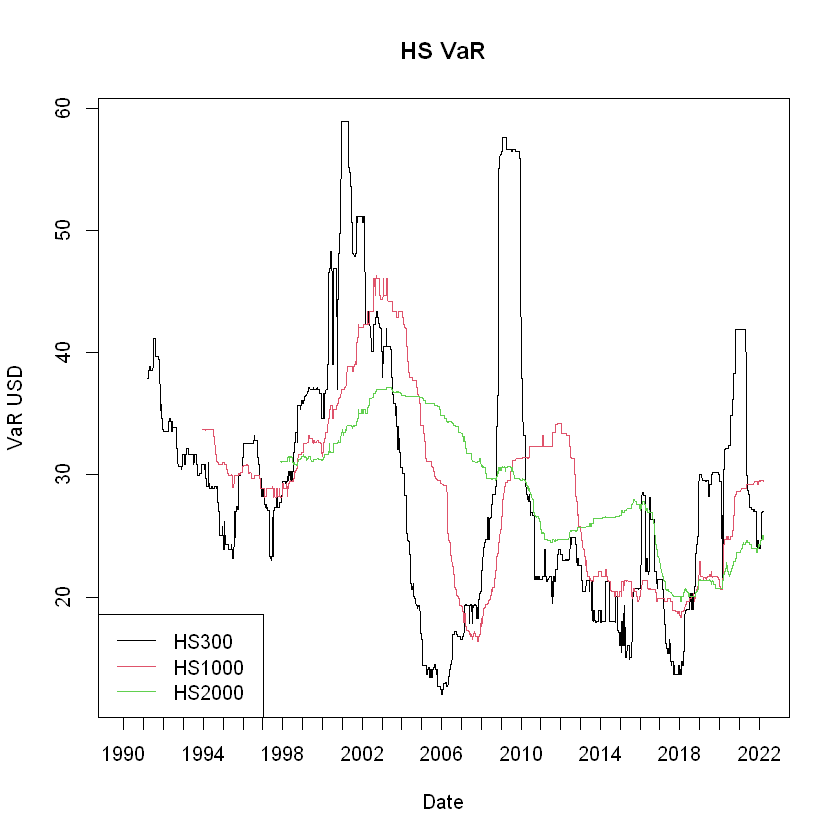## EWMA VaR¶

We can use the estimated conditional volatility from an EWMA model, $\hat{\sigma}_t$, to forecast VaR:

$$\textrm{VaR}_t(p) = -\hat{\sigma}_t F_{R}^{-1}(p)\ \vartheta$$

Where $p$ is the specified VaR probability, $F_{R}^{-1}(\cdot)$ is the inverse of the cdf of returns, and $\vartheta$ is the portfolio value.

The minimum estimation window to implement our EWMA is 30 days. In our implementation, this will be the Burn time. We initialize the EWMA model with the sample covariance as the value for the first time period. The effect of a given conditional covariance from a past period quickly dies out as time passes, because $\lambda^n \rightarrow 0$ as $n \rightarrow \infty$, so the effect of initializing the EWMA matrix with the unconditional covariance will not be a problem after a few time periods. The rule of thumb is to burn the first $W_E$ observations, usually taken as 30 days.

Let's implement this model to the Microsoft returns:

In :
# Implementing EWMA VaR

# Specifying the parameters for VaR
p <- 0.05
portfolio_value <- 1000

# Specifying the parameters for EWMA
lambda <- 0.94
n <- length(y)
BurnTime <- 30

# Vector to store estimated conditional volatility
EWMA_Variance <- rep(NA, length = n)

# First value is the sample variance
EWMA_Variance <- var(y)

# See the vector

1. 0.000400008253055721
2. <NA>
3. <NA>
4. <NA>
5. <NA>
6. <NA>

Now that we have created the EWMA vector and used the sample variance to fill the first value, we can use a for loop to run the EWMA model:

In :
# Run the EWMA model using a for loop
for (i in 2:n) {
EWMA_Variance[i] <- lambda * EWMA_Variance[i-1] + (1-lambda) * y[i-1]^2
}


Since we initialized the EWMA matrix using the unconditional sample variance, we burn the data in the estimation window $W_E = 30$.

In :
# Replacing the data in the estimation window to NA
EWMA_Variance[1:BurnTime] <- NA


We can plot the square root of EWMA_Variance to see our estimation for conditional volatility:

In :
# Plot estimation for conditional volatility
EWMA_cond_volatility <- sqrt(EWMA_Variance)
plot(dates, EWMA_cond_volatility, type = "l", main = "EWMA Conditional Volatility",
las = 1, col = "red", xlab = "Date", ylab = "EWMA Cond. Volatility")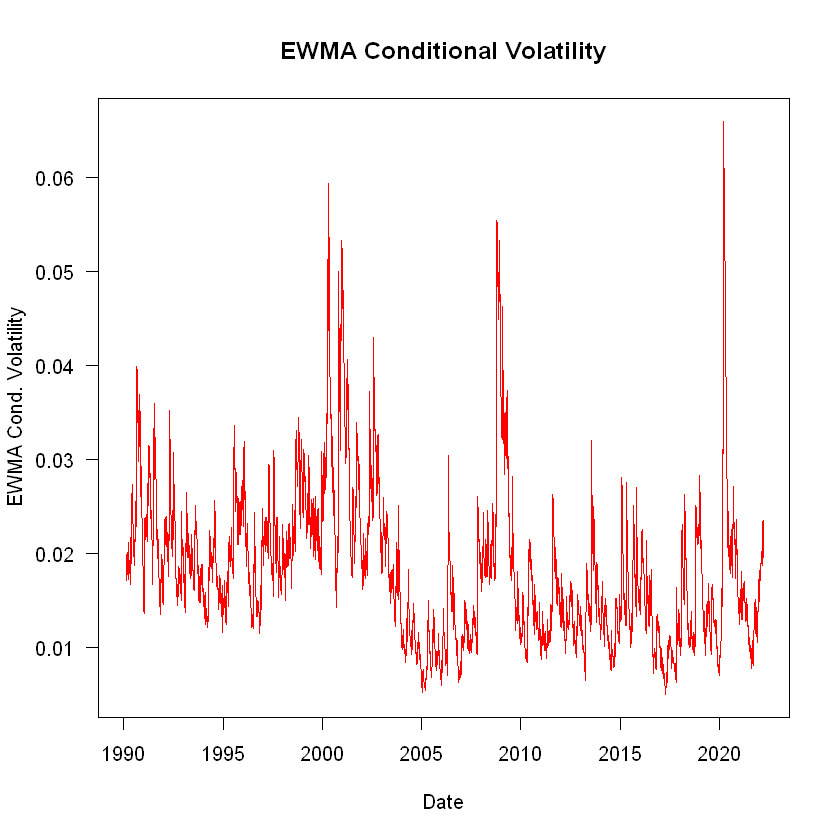To forecast VaR, we need to assume a conditional distribution for the returns. We will assume conditional normality:

In :
# Implementing the VaR forecast
EWMA_VaR <- -qnorm(p) * EWMA_cond_volatility * portfolio_value

# Plotting it
plot(dates, EWMA_VaR, type = "l", main = "EWMA VaR",
las = 1, col = "red", xlab = "Date", ylab = "USD")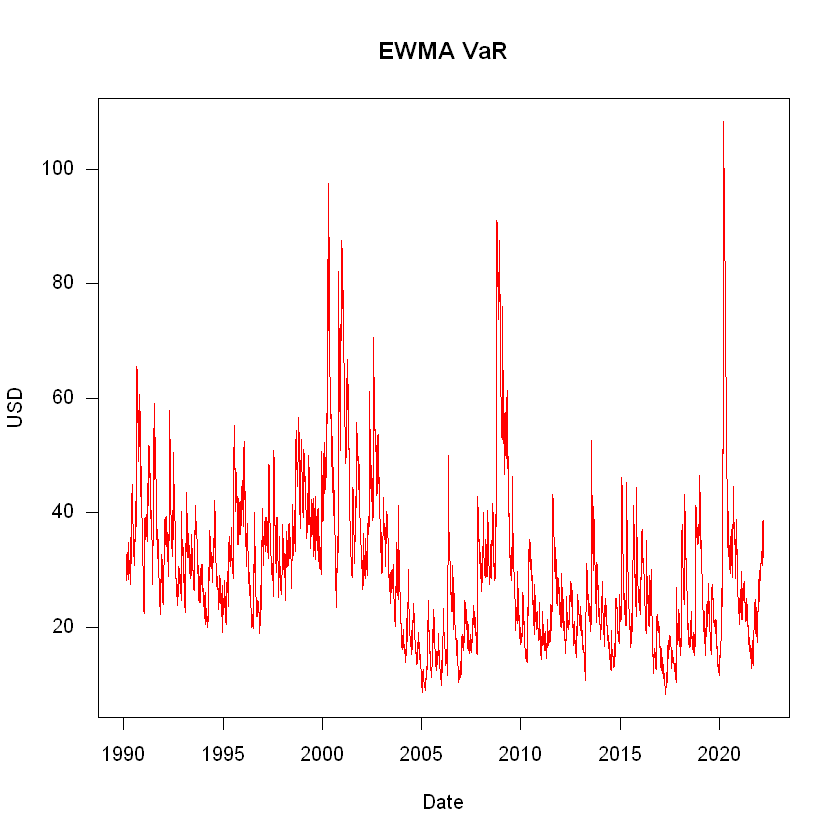We will continue working with these models in the next seminar, so let's save the VaR forecasts for the historical simulation and EWMA in a dataframe called VaR:

In :
# Storing the data
VaR <- cbind(HS_VaR, EWMA_VaR)
VaR <- as.data.frame(VaR)
save(VaR, file = "VaR.RData")


## Recap¶

In this seminar we have covered:

• Review questions on risk measures
• Univariate historical simulation
• Multivariate historical simulation
• VaR forecasting with historical simulation
• Implementing historical simulation with different estimation windows
• Implemented EWMA VaR

Some new functions used:

• segments()
• colMeans()

For more discussion on the material covered in this seminar, refer to Chapter 4: Risk measures and Chapter 5: Implementing risk forecasts on Financial Risk Forecasting by Jon Danielsson.

Acknowledgements: Thanks to Alvaro Aguirre and Yuyang Lin for creating these notebooks